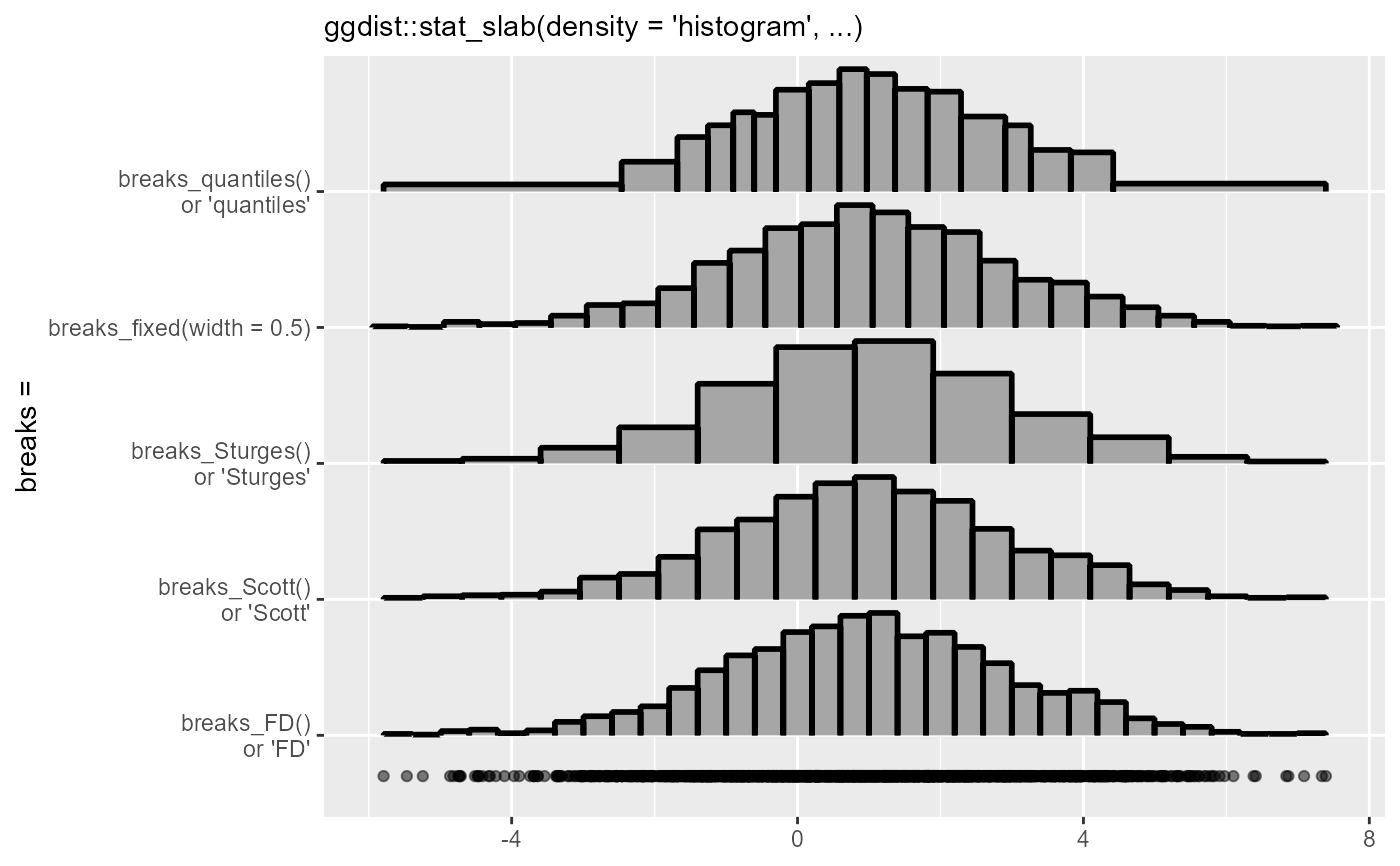Methods for determining breaks (bins) in histograms, as used in the breaks argument to density_histogram(). Supports automatic partial function application.

breaks_fixed(x, weights = NULL, width = 1)

breaks_Sturges(x, weights = NULL)

breaks_Scott(x, weights = NULL)

breaks_FD(x, weights = NULL, digits = 5)

## Arguments

x

A numeric vector giving a sample.

weights

A numeric vector of length(x) giving sample weights.

width

For breaks_fixed(), the desired bin width.

digits

Number of significant digits to keep when rounding in the Freedman-Diaconis algorithm (breaks_FD()). For an explanation of this parameter, see the documentation of the corresponding parameter in grDevices::nclass.FD().

## Value

Either a single number (giving the number of bins) or a vector giving the edges between bins.

## Details

These functions take a sample and its weights and return a valuable suitable for the breaks argument to density_histogram() that will determine the histogram breaks.

• breaks_fixed() allows you to manually specify a fixed bin width.

• breaks_Sturges(), breaks_Scott(), and breaks_FD() implement weighted versions of the corresponding base functions. See nclass.Sturges(), nclass.scott(), and nclass.FD().

density_histogram(), align

## Examples

library(ggplot2)

set.seed(1234)
x = rnorm(200, 1, 2)

# Let's compare the different break-selection algorithms on this data:
ggplot(data.frame(x), aes(x)) +
stat_slab(
aes(y = "breaks_fixed(width = 0.5)"),
density = "histogram",
breaks = breaks_fixed(width = 0.5),
outline_bars = TRUE,
color = "black",
) +
stat_slab(
aes(y = "breaks_Sturges()\nor 'Sturges'"),
density = "histogram",
breaks = "Sturges",
outline_bars = TRUE,
color = "black",
) +
stat_slab(
aes(y = "breaks_Scott()\nor 'Scott'"),
density = "histogram",
breaks = "Scott",
outline_bars = TRUE,
color = "black",
) +
stat_slab(
aes(y = "breaks_FD()\nor 'FD'"),
density = "histogram",
breaks = "FD",
outline_bars = TRUE,
color = "black",
) +
geom_point(aes(y = 0.7), alpha = 0.5) +
labs(
subtitle = "ggdist::stat_slab(density = 'histogram', ...)",
y = "breaks =",
x = NULL
)